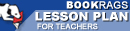Lesson Plans

# Daily Lessons for Teaching Journey Through Genius: The Great Theorems of Mathematics

This set of Lesson Plans consists of approximately 142 pages of tests, essay questions, lessons, and other teaching materials.
 View a FREE sample## Lesson 1 (from Hippocrates' Quadrature of the Lune)

### Objective

Hippocrates's Quadrature of the Lune

In this section the author began with a brief overview of early mathematics. He explained that one of the first ancient mathematicians to study geometric relationships was Thales. In this lesson students will examine the basic understanding of geometry before Thales and how Pythagoras advanced geometry beyond the ideas of Thales and constructed the Pythagorean Theorem.

### Lesson

1) Group discussion: Assign students to groups of 3-4 students, and designate a group leader. Instruct the group leaders to give each group member one of the following questions to answer. What basic geometric principles did people understand before Thales? What was understood about right triangles before Thales? How did Pythagoras describe triangles? Why was the Pythagorean Theorem considered a logical proof? When students complete their question, each group leader should organize their group to give a small presentation to summarize their answers.

2) Class discussion...

(read more Daily Lessons)

 This section contains 6,733 words (approx. 23 pages at 300 words per page) View a FREE sampleCopyrightsJourney Through Genius: The Great Theorems of Mathematics from BookRags. (c)2019 BookRags, Inc. All rights reserved.
Follow Us on Facebook